### Error Spending Methods

For each sequential design, theanderrors spent at each stage can be computed from the boundary values. For example, for a K-stage design with an upper alternative hypothesis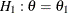and early stopping to reject the null hypothesis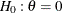, the boundary values in a standardized Z scale are the uppercritical values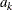,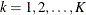. At each interim stage, the null hypothesisis rejected if the observed standardized Z statistic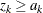. Otherwise, the process continues to the next stage. At the final stage, the hypothesis is rejected if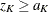. Otherwise, the null hypothesis is accepted.

The boundary valuesare derived such that the overall Type I error probability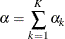where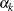is thespending at stage k. That is, at stage 1,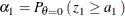At a subsequent stage k,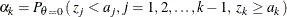Since each design can be uniquely identified by theanderrors spent at each stage, a design can then be derived by specifying theanderrors to be used at each stage. The error spending method (Lan and DeMets, 1983) distributes the error to be used at each stage and then derives the boundary values. Numerous forms of the error spending function are available. Kim and DeMets (1987) examine the functions,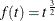, and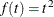, where t is the information fraction. Jennison and Turnbull (2000, p. 148) generalize these functions to the power functions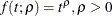.

The ERRFUNCPOC option uses the cumulative error spending function (Lan and DeMets, 1983)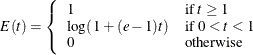With a specified error ofor, the cumulative error spending at stage k is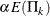or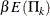, where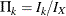is the information fraction at stage k. The method produces boundaries similar to those produced with Pocock’s method.

The ERRFUNCOBF option uses the cumulative error spending function (Lan and DeMets, 1983)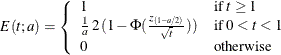where a is eitherfor thespending function orfor thespending function. That is, with a specified error ofor, the cumulative error spending at stage k is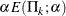or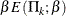. The method produces boundaries similar to those produced with the O’Brien-Fleming method.

The ERRFUNCGAMMA option uses the gamma cumulative error spending function (Hwang, Shih, and DeCani, 1990)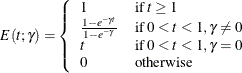whereis the parameterspecified in the GAMMA= option. That is, with a specified error ofor, the cumulative error spending at stage k is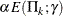or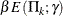.

The ERRFUNCPOW option uses the cumulative error spending function (Jennison and Turnbull, 2000, p. 148)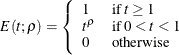where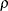is the power parameter specified in the RHO= option. That is, with a specified error ofor, the cumulative error spending at stage k is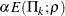or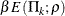.

Error spending methods derive boundary values at each stage sequentially and require much more computation than other types of methods for group sequential trials with a large number of stages, especially for a two-sided asymmetric design with early stopping to accept, or to reject or accept.

Note that for a two-sided design with the STOP=BOTH or STOP=ACCEPT option, at each interim stage, the SEQDESIGN procedure first produces the lower and upperboundary values based on the one-sidedspending. If the lowerboundary value is greater than or equal to its corresponding upperboundary value, there is no early stopping to accept the null hypothesis at this stage, and the correspondingspending is distributed proportionally to the remaining stages.

For the error spending functions not available in the SEQDESIGN procedure, you can first compute the corresponding error spending at each stage explicitly, then use the SEQDESIGN procedure with the ERRSPEND= option to specify these errors directly.

For example, if the information levels are equally spaced in a five-stage design, the option ERRFUNCPOW (RHO=2) produces relative cumulative errors of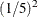,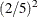,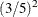,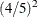, and 1. This is equivalent to using the option ERRSPEND (1 4 9 16 25).

A one-sided error spending design is illustrated in Example 83.8 and a two-sided asymmetric error spending design is illustrated in Example 83.11.